Updated date:

# Use the Rank and Percentile Tool from the Analysis ToolPak in Excel 2007 and Excel 2010 to Create Ranking Tables

Robbie mostly writes about Skyrim but also occasionally sheds light on the oddities of Microsoft applications such as Excel and Outlook.

## Introduction

In this article, I will be investigating the Rank and Percentile Tool.

Ranking allows you to rank all your data in order from highest to lowest and Percentile allows you to easily categorise your data into percentiles such as the top 25%, top 50% etc.

To illustrate how to use this tool, I will work through an example. In my example, I have a class of 30 students. I have just got their latest results and want to rank them and assign them grades based on their ranking within the class. The top 10% get an A, the next 20% get a B, the next 20% get a C and the remainder get a D grade.

My ultimate goal is to end up with a table such as the one shown below with as little manual work as possible: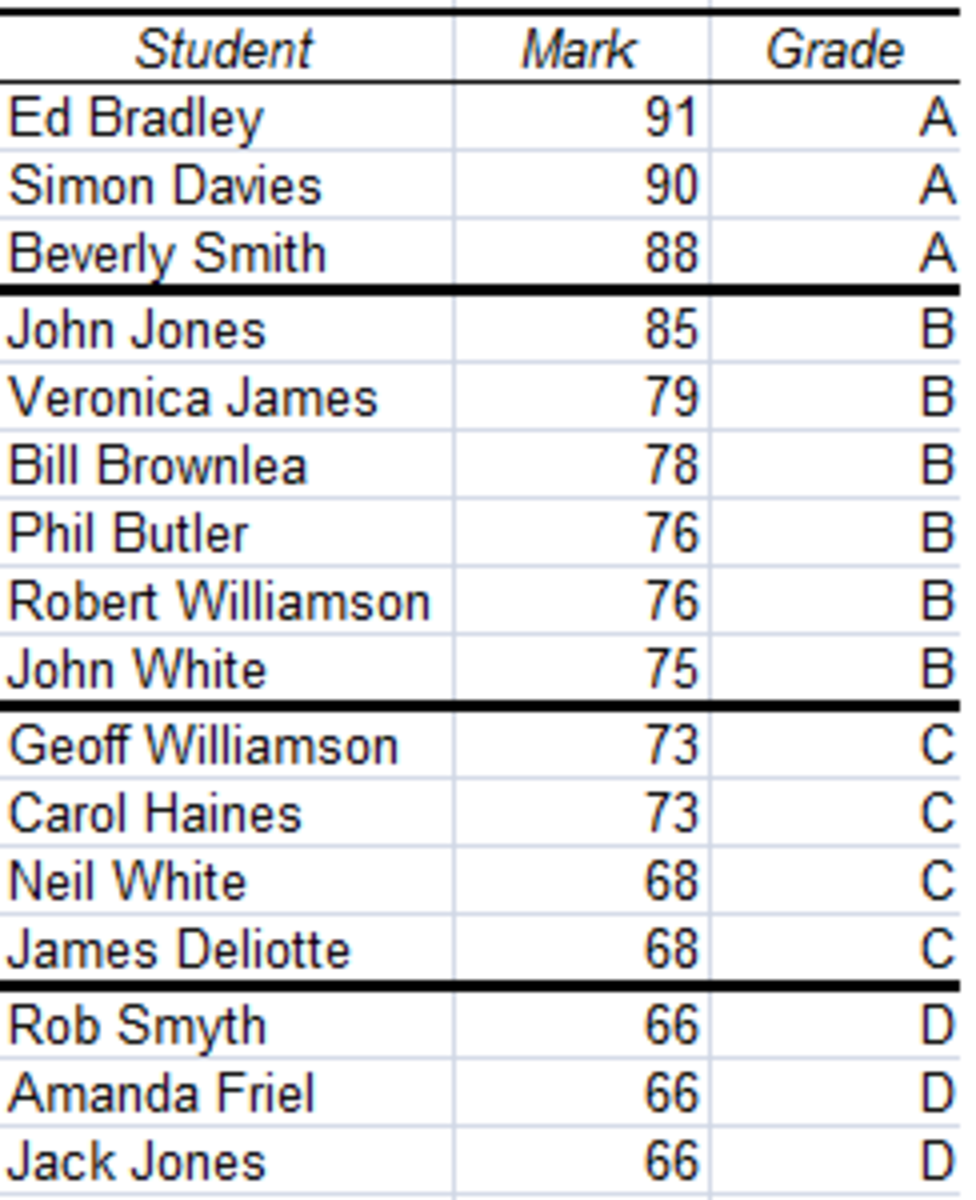Ranking Table created using the Rank and Percentile Tool from the Analysis Toolpak in Excel 2007 and Excel 2010.http://robbiecwilson.hubpages.com/

In conjunction with the Rank and Percentile tool, I will use the MATCH and INDEX functions to assign the student names to the raw data created by the tool. I will then use the IF function to automatically assign a grade to them based on their result as well.

## Adding the Analysis ToolPak in Excel 2007 and Excel 2010

Before using the tool, we need to ensure that the Analysis ToolPak is present in Excel. The ToolPak can be found on the Data tab, in the Analysis group and is accessed via the Data Analysis button.

## Using the Rank and Percentile Tool in Excel 2007 and Excel 2010

Now that we have added the Analysis ToolPak, select the Data Analysis button and select Rank and Percentile as shown below.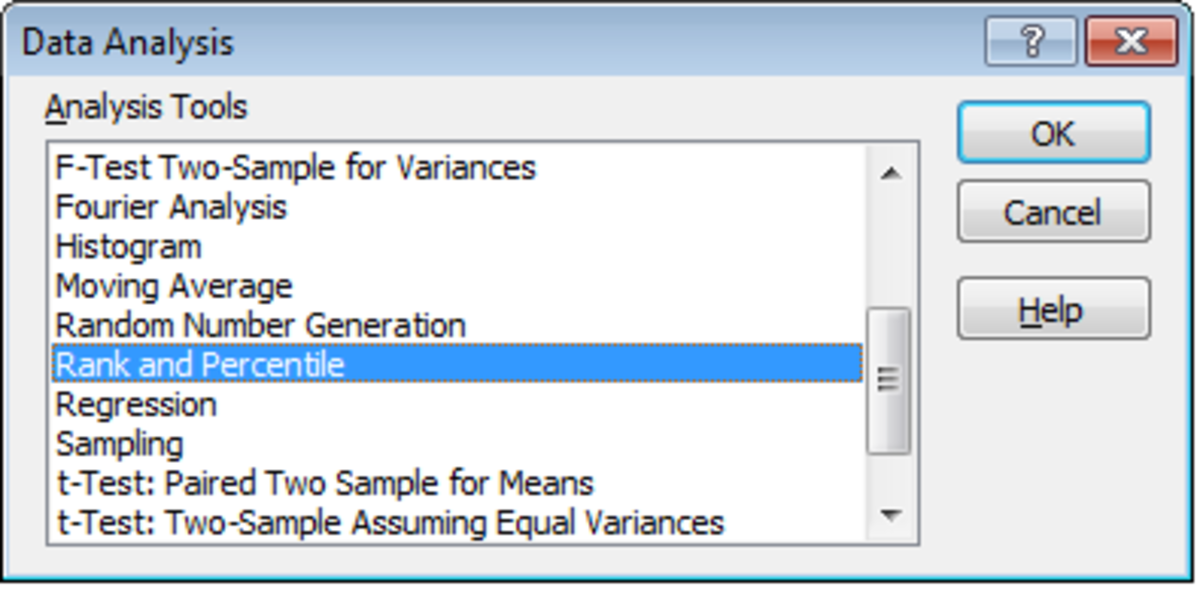Selecting the Rank and Percentile Tool in the Analysis ToolPak using Excel 2007 and Excel 2010.http://robbiecwilson.hubpages.com/

The tool itself is quite straightforward and easy to use:

• First select the Input Range
• If your data is in columns, leave Grouped By as Columns (otherwise select Rows)
• If you have labels for your columns, then select Labels in first row
• Lastly, select your Output options, select Output Range to place it in a specific place on your worksheet or select New Worksheet Ply or New Workbook depending on your preference (we placed the tool’s results next to the original data in the example we are working through today)
• Click OK and Excel will create your Rank and Percentile table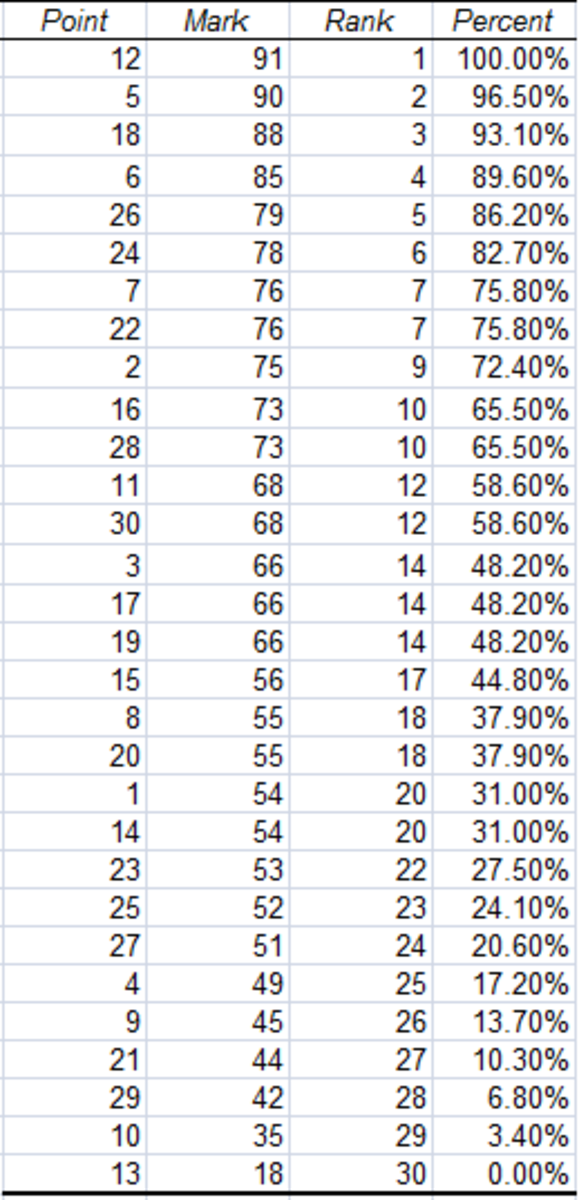Output of the Rank and Percentile Tool, created using the Rank and Percentile Tool from the Analysis Toolpak in Excel 2007 and Excel 2010.http://robbiecwilson.hubpages.com/

You can see the output above from the tool. Excel has ranked every student and given them their exact percentile (the Percent column), however, rather than using the student's names, there is a column called Point, which is the row number of each student. To turn the Point column into a column containing the student’s name, I need to turn to the MATCH and INDEX function.

## Using the MATCH and INDEX Function in Excel 2007 and Excel 2010 to Convert the Results in the Point Column Created by the Rank and Percentile Tool to the Actual

These two functions work in tandem to convert the results in the Point column to the student’s name.

First:

• The MATCH function looks for the number in the Point column and then finds its exact match in the column labelled Student No.
• INDEX then looks for the cell adjacent to the result that MATCH found and returns the student’s name.

In the figure below, you can see the formula that turns 12 in the Point column to our top student (Ed Bradley)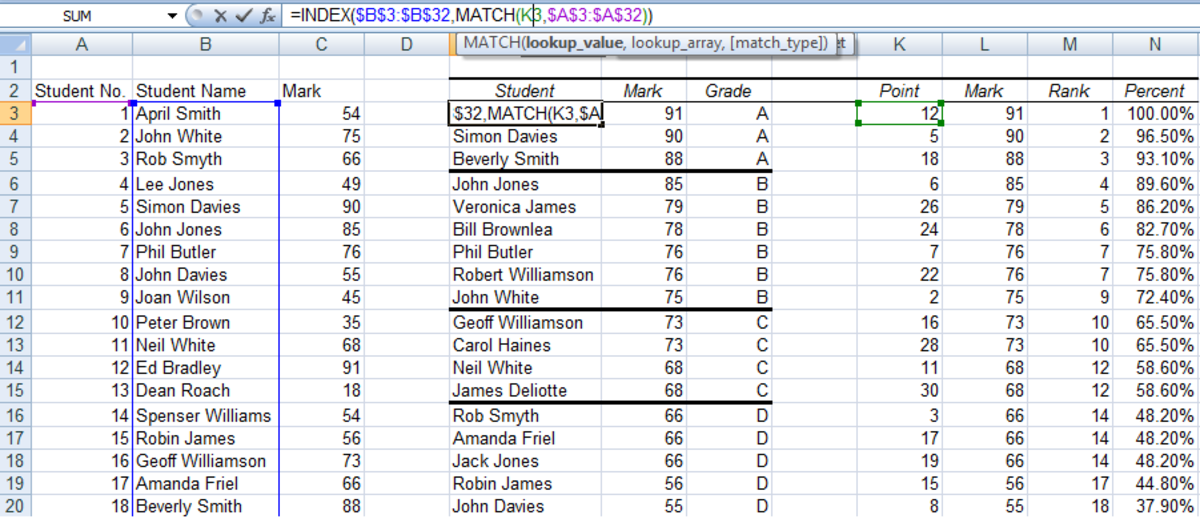The MATCH and INDEX function matching the results with the student's name in Excel 2007 and Excel 2010.http://robbiecwilson.hubpages.com/

The entire formula is

=INDEX(\$B\$3:\$B\$32,MATCH(F5,\$A\$3:\$A\$32))

It is easier to understand broken down into its component parts, MATCH and INDEX

• The MATCH function looks for the contents of F5 (12) in the range A3 to A32

MATCH(F5,\$A\$3:\$A\$32))

• The INDEX function then looks in the range B3 to B32 for the cell adjacent to the cell found by MATCH

So, MATCH finds 12 in cell A14 and INDEX finds Ed Bradley in the cell adjacent to A14

Note: the \$’s added to the cell references tells Excel 2007 and Excel 2010 that these cell references do not change even when the formula is copied to other cells (known as absolute cell references). This prevents Excel from adding one to each cell reference each time you copy it (the formula would become =INDEX(B4:B33,MATCH(F4,A4:A33)) rather than =INDEX(\$B\$3:\$B\$32,MATCH(F4,\$A\$3:\$A\$32)) which may otherwise provide a potentially incorrect result).

I placed this formula in the column to the right of the Point column to make it easier to complete our final table with the column header of Student.

## Completing our table ranking our students in Excel 2007 and Excel 2010

Now that we have a column containing our students names, to complete the table:

• Hide the Point and Rank columns (do not delete them or the formulas will break)
• Copy the Percent column and place it to the right of the original column
• Change the name of the original Percent column to Grade

Remember that we wanted to do this as automatically as possible. To assign our students a grade, we will use an IF statement as shown below:

=IF(K3<50%, "D", IF(K3>90%, "A", IF(K3>70%,"B", IF(K3>50%, "C"))))

This formula looks at the Percent column and looks at the percent and then assigns a letter based on the result it finds.

Note: The order is vitally important, if we did it in the order C, B, A then we would end up with only D and C grades as Excel would look at all numbers above 50% and give them a C and the formula would end.

• Copy this formula to the remainder of the Grade column.

Now we have our completed table, which looks like the figure below: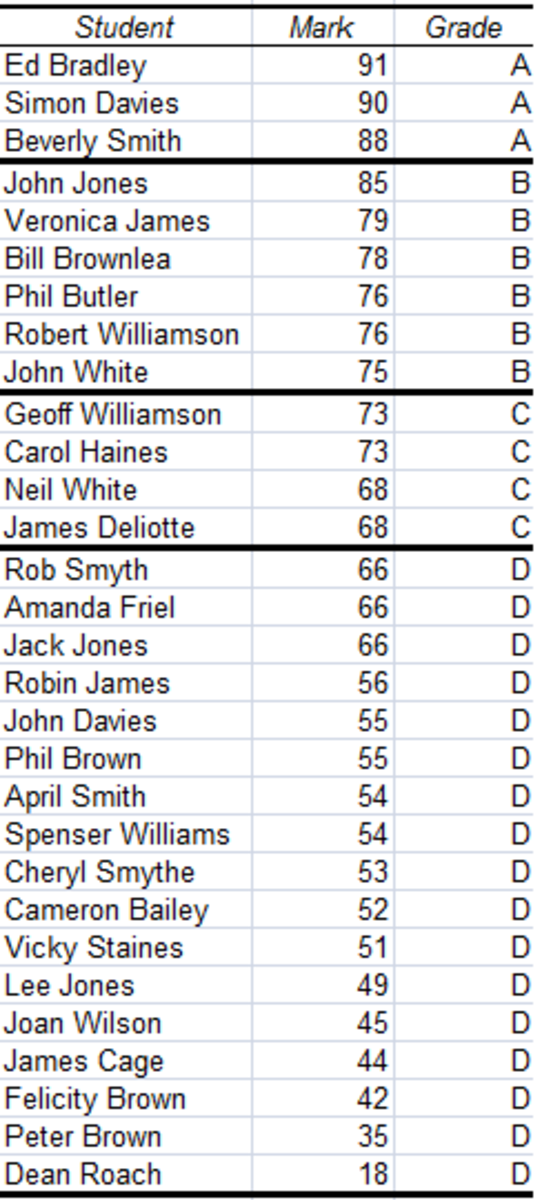Completed ranking table created using the Rank and Percentile Tool, MATCH, INDEX and IF functions in Excel 2007 and Excel 2010.http://robbiecwilson.hubpages.com/

## Conclusion

The Rank and Percentile Tool from the Analysis ToolPak which is available in Excel 2007 and Excel 2010 allows us to work with data and rank it and then have Excel calculate its percentile.

In my example today, I took my (fictional) class of students and ranked them according to their test results. I then gave them a grade depending on where they sat ranked against their peers.

To accomplish this, I not only used the Rank and Percentile Tool, but I also used the MATCH and INDEX functions to convert the results so that the name of the student appeared next to their grade, and then also used the IF command to automatically assign them their grade.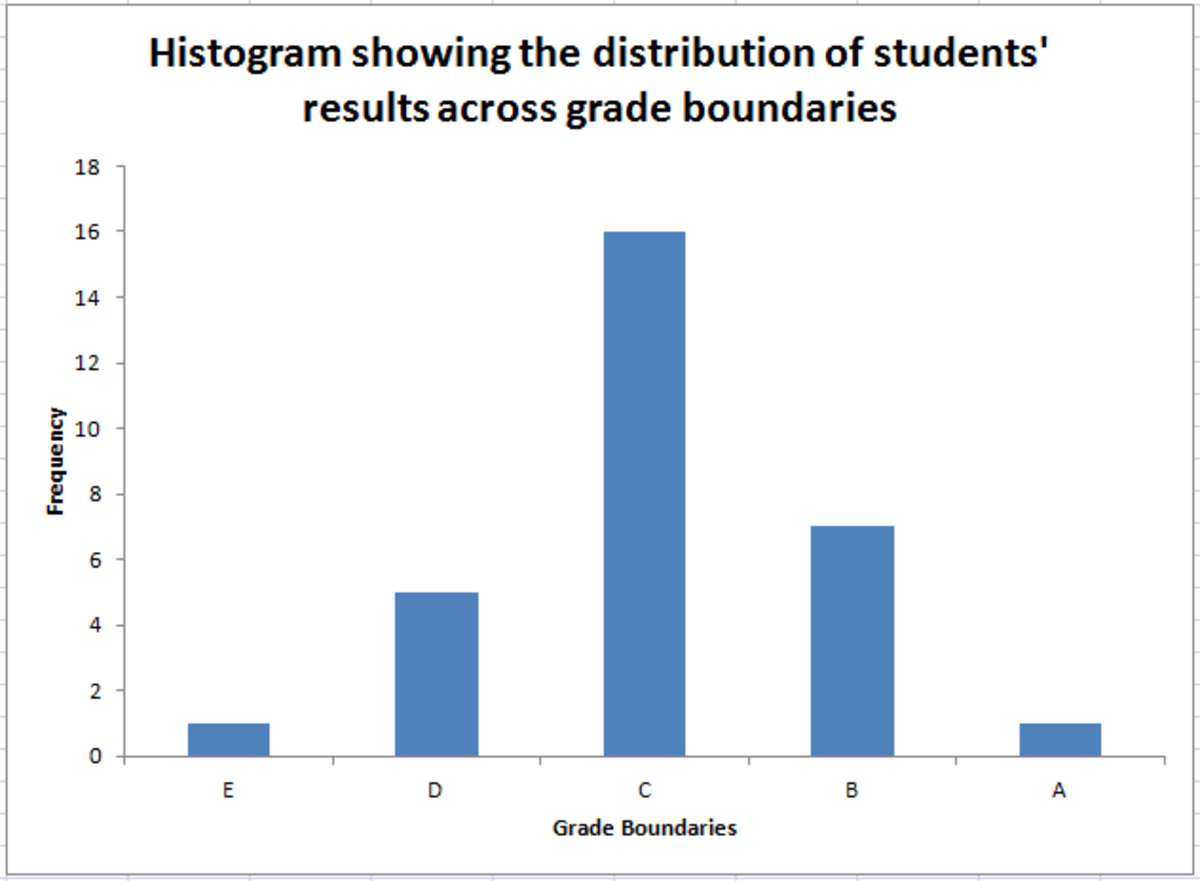Example of a Histogram, created using the Histogram Tool from the Analysis Toolpak in Excel 2007 and Excel 2010.http://robbiecwilson.hubpages.com/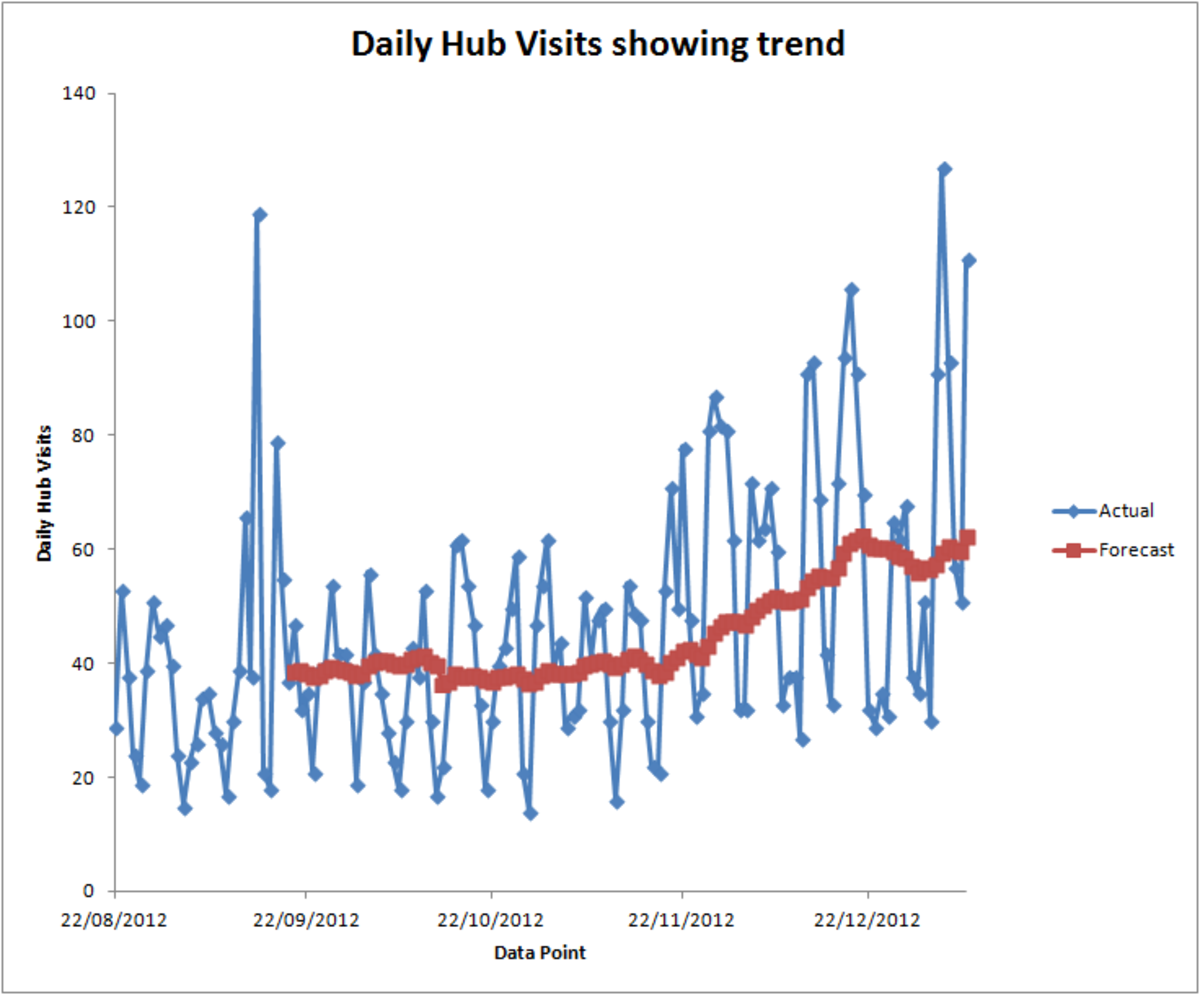Example of a Moving Average, created using the Moving Average Tool from the Analysis Toolpak in Excel 2007 and Excel 2010.http://robbiecwilson.hubpages.com/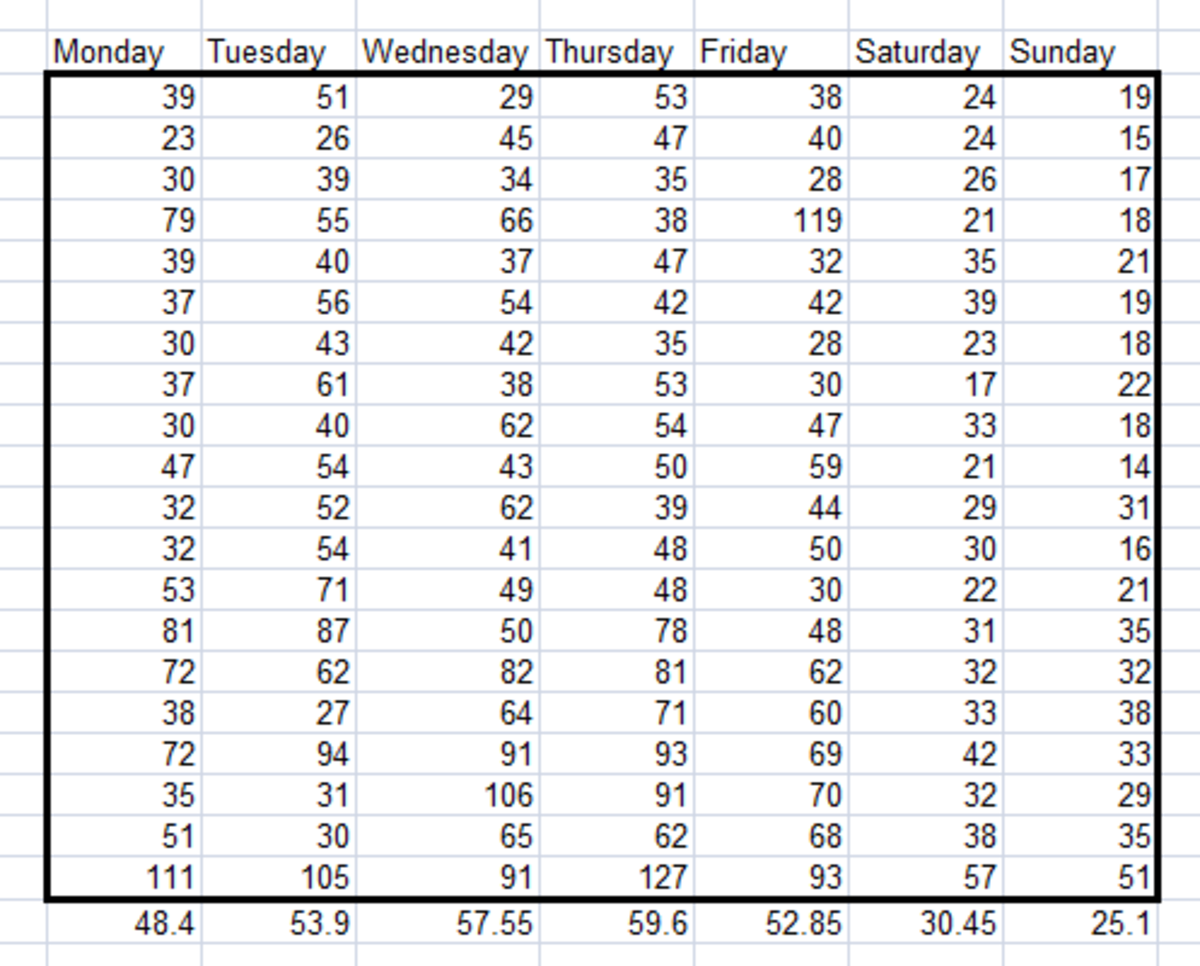Example of a table showing daily variations, created using the Sampling Tool from the Analysis Toolpak in Excel 2007 and Excel 2010.http://robbiecwilson.hubpages.com/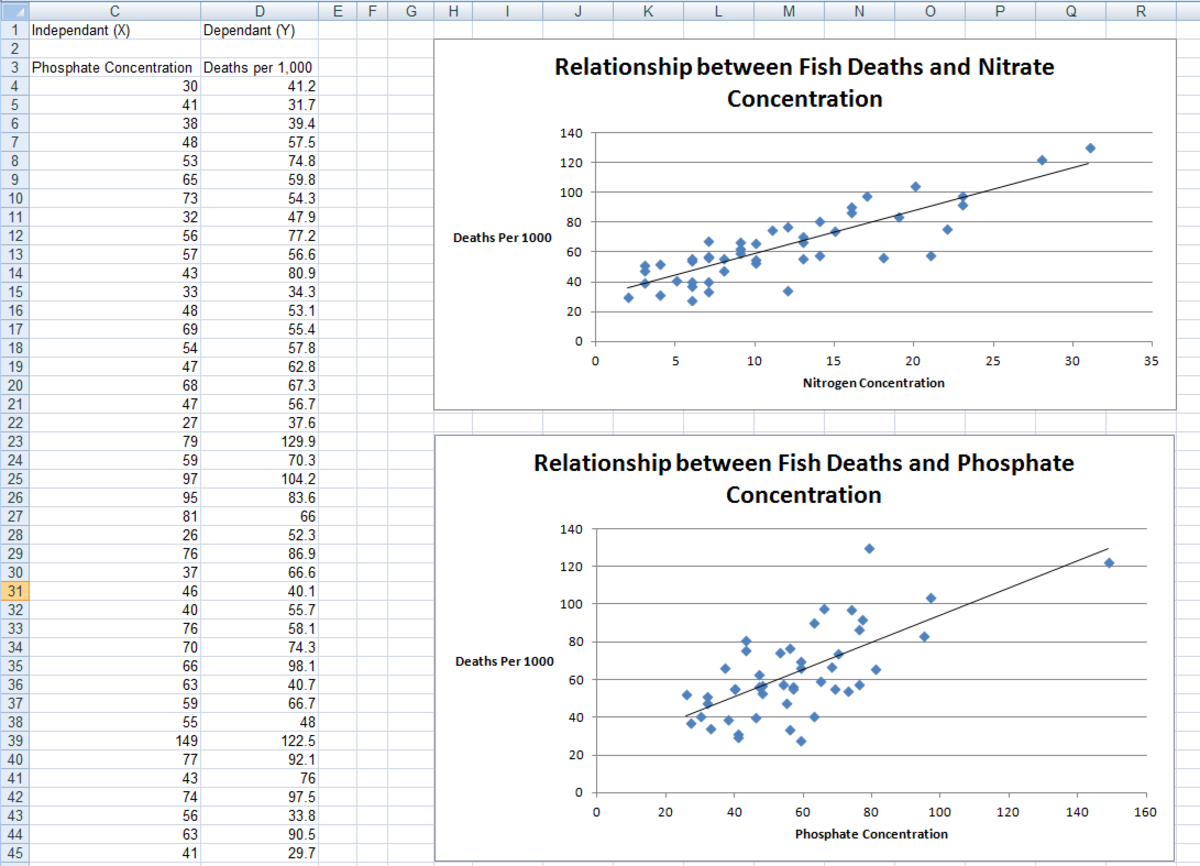Example of a Regression, created using the Regression Tool from the Analysis Toolpak in Excel 2007 and Excel 2010.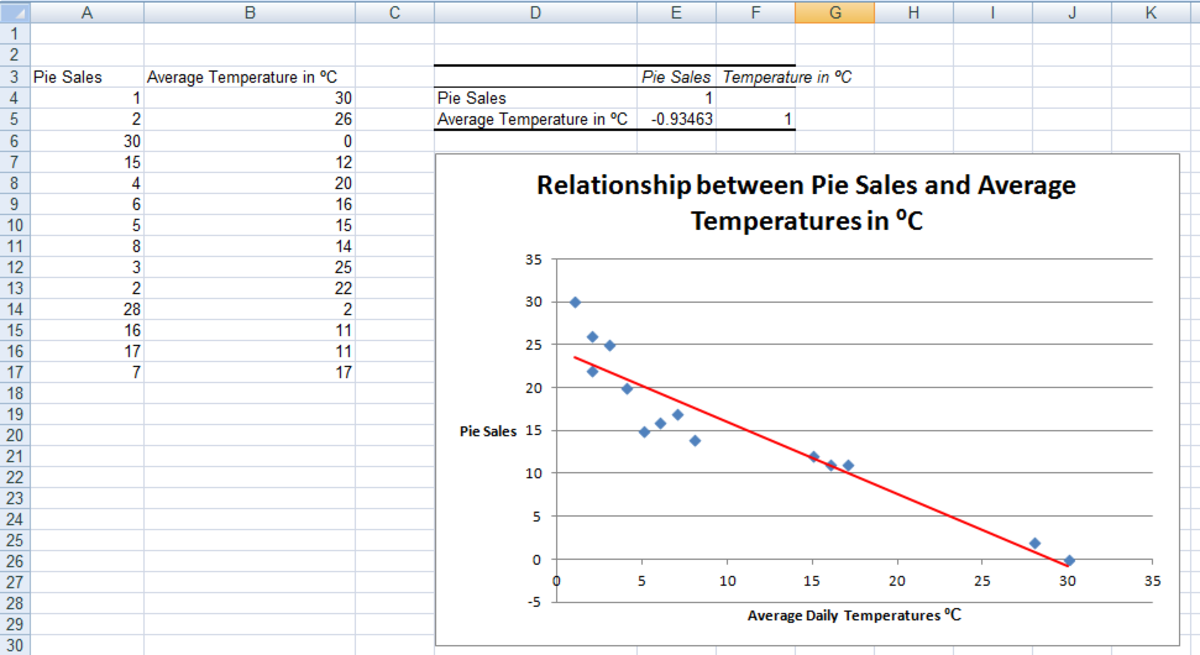Example of a Correlation, created using the Correlation Tool from the Analysis Toolpak in Excel 2007 and Excel 2010.